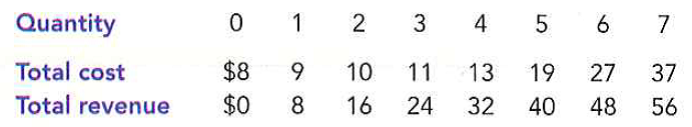Problem

# Consider total cost and total revenue given in the following table: a. Calculat...

Consider total cost and total revenue given in the following table:a. Calculate profit for each quantity. How much should the firm produce to maximize profit?

b. Calculate marginal revenue and marginal cost for each quantity. Graph them. (Hint: Put the points between whole numbers., For example, the marginal cost between 2 and 3 should be graphed at 272.) At what quantity do these curves cross? How does this relate to your answer to part (a)?

c. Can you tell whether this firm is in a competitive industry? If so, can you tell whether the industry is in a long-run equilibrium?

#### Step-by-Step Solution

Request Professional Solution

#### Request solution!

We need 10 more requests to produce this problem solution. Share with your friends to get the problem solution faster!

0 /10 have requested this problem solution

Once 10 people have made a request, the problem solutions will be available in 1-2 days.
All students who have requested the problem solution will be notified once they are available.